# Python pop() Function

The pop() function in Python is used to remove an item from the list. For example:

```mylist = [10, 40, 50, 60, 30, 32]

mylist.pop()
print(mylist)

mylist.pop(3)
print(mylist)```

The output produced by above Python program, demonstrating the pop() function is given below:

```[10, 40, 50, 60, 30]
[10, 40, 50, 30]```

Note: If you'll not provide any argument to the pop(), then the last element will get deleted. And if you'll provide any argument to the pop(), then the argument get considered as the index. And the element available at that index, will get deleted.

The pop() function can also be used to remove an item from the dictionary. For example:

```mydict = {"Name": "Logan", "Region": "Colorado"}
mydict.pop("Region")
print(mydict)```

Following is the output produced by this program:

`{'Name': 'Logan'}`

In case of dictionary, the key value must be specified as the argument of pop() to remove the item with that specified key.

## Python pop() Function Syntax

The syntax to use pop() function in Python is given below. This syntax is used when working with list:

`listName.pop(position)`

where position is the index number of the element, that is going to remove. The position argument is optional. The default value of position argument is -1. The index -1 refers to the last element. Therefore, the last element will get deleted when we did not provide any argument to pop.

Note: The pop() function returns the removed item.

Here is another syntax of pop() function, when working with dictionary:

`mydict.pop(keyname, defaultValue)`

The keyname is required, whereas the defaultValue is optional. The defaultValue is used to avoid raising the error, when the specified keyname is not available in the dictionary.

Note: Don't think much about the syntax, you'll learn all about it, later using the example program given below.

## Python pop() Function Example

This section is divided into two category. One contains the example of pop() function, when working with list, whereas the second contains the example of pop() while working with dictionary. So let's start with the list first.

### Python pop() Function Example - For List

Here is a simple example uses pop() function to remove an element from the list:

```x = [12, 34, 54, 65, 7, 8]
print("The list is:")
print(x)

print("\nEnter the Position (Index No.) of Element to Delete: ", end="")
pos = int(input())
x.pop(pos)
print("\nNow the list is:")
print(x)```

The sample run of above program, with user input 4 as index number to delete the element available at index 4 is shown in the snapshot given below: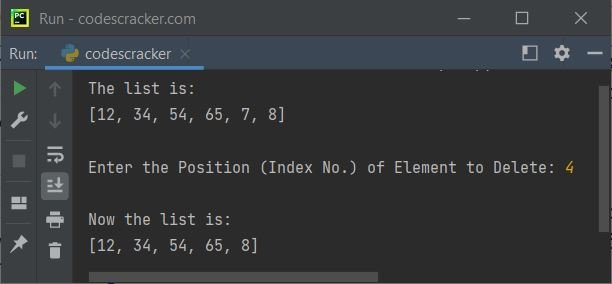Still the program is not perfect, because when user enters the position that is greater than the length of the list, then the program try to pop the element available at index, that does not exist in the list. And in that case, there will some error gets produced like shown in the snapshot of the sample run given below: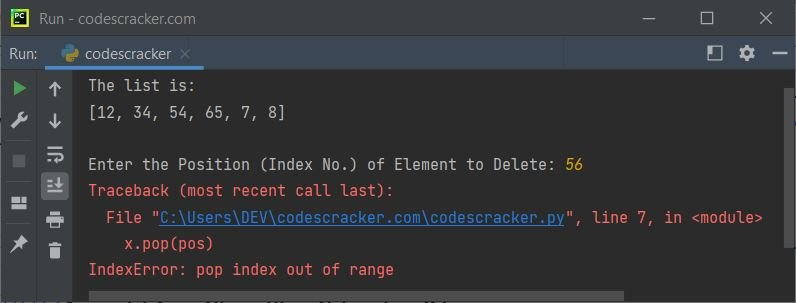Now this can be handled, either using the try and except block or by checking whether the entered index number is less than the length of the list or not. The second way is dealt with the second program given below. But for now, let's create a program, that uses the first way to handle the error raised by pop() function in Python:

```x = [12, 34, 54, 65, 7, 8]
print("The list is:")
print(x)

print("\nEnter the Position (Index No.) of Element to Delete: ", end="")
pos = int(input())
try:
x.pop(pos)
print("\nNow the list is:")
print(x)
except IndexError:
print("\nInvalid Index Number!")```

Now if you try to enter some invalid index number, then the program executes the statement(s) available inside the except block. Here is its sample run with same user input as provided in previous sample run: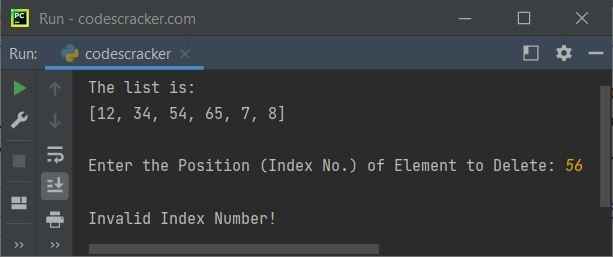Here is the modified version of previous program, allows user to choose, whether to pop the last element or to pop the element at particular index:

```print("Enter the size of list: ", end="")
tot = int(input())
print("Enter", tot, "elements for the list: ", end="")
x = []
for i in range(tot):
val = input()
x.append(val)

print("\nThe list is:")
for i in range(tot):
print(x[i], end=" ")

print("\n\n1. Remove Last Element")
print("2. Remove Element with Particular Index")
print("Enter Your Choice (1 or 2): ", end="")
choice = input()
if choice == '1':
x.pop()
print("\nNow the list is:")
for i in range(len(x)):
print(x[i], end=" ")
elif choice == '2':
print("\nEnter the Index Number: ", end="")
pos = int(input())
if pos < tot:
removed = x.pop(pos)
print("\nThe element at index", pos, "is deleted!")
print("The deleted element is:", removed)
print("\nNow the list is:")
for i in range(len(x)):
print(x[i], end=" ")
else:
print("\nInvalid Input!")
else:
print("\nInvalid Input!")```

The snapshot given below shows the sample run of above program with user input 8 as size of list, 12, 23, 34, 45, 56, 67, 78, 89 as eight elements, 2 as choice, 5 as index number to delete the element: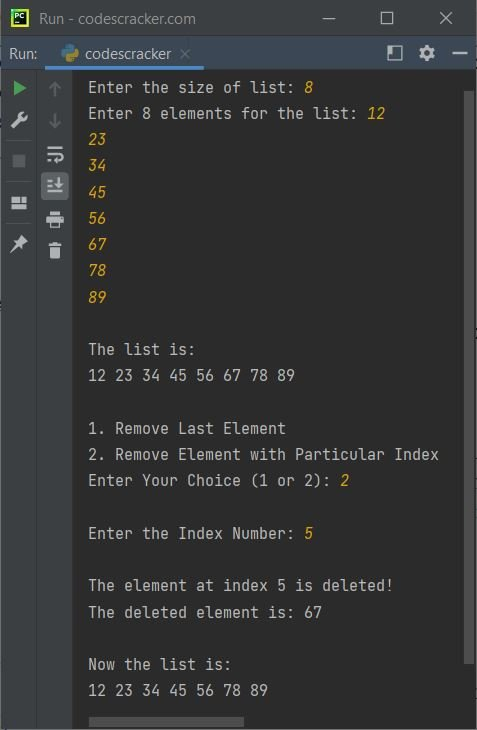### Python pop() Function Example - For Dictionary

Here is an example program uses pop() function to pop or remove an item from the dictionary specified by the key of the item, by user at run-time of the program:

```x = {"Day": "Sat", "Month": "Nov", "Year": "2021"}
print("The dictionary is: ")
print(x)

print("\nEnter the key to pop: ", end="")
key = input()
x.pop(key)
print("\nNow the dictionary is: ")
print(x)```

The snapshot given below shows the sample run with user input Day as key: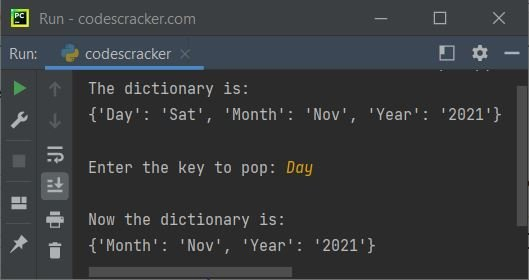Similar to previous section that uses pop() while working with list. Below is another program, that can be considered as the modified version of above program.

This is the last program of this article, created to show you, how an item with particular or specified key will get deleted from the dictionary, using the pop() function:

```print("Enter the dictionary size: ", end="")
tot = int(input())
print("Enter", tot, "Items for the dictionary\n")
mydict = {}
for i in range(tot):
print("Enter Key and Value of Item No.", i+1, ": ", end="")
key = input()
value = input()
mydict.update({key: value})

print("\nThe dictionary is: ")
print(mydict)

print("\nEnter the Key to Delete the Particular Item: ", end="")
key = input()
try:
removed = mydict.pop(key)
print("\nThe item with key \"", key, "\" gets deleted.")
print("\nNow the dictionary is: ")
print(mydict)
except KeyError:
print("\nInvalid Key!")```

The sample run with user input 3 as size of dictionary, Name and Ryan as key and value for first item, Region and Texas as key and value for second item, Course and Data Science as key and value for the third and last item, and finally Region as key to delete the item, is shown in the snapshot given below: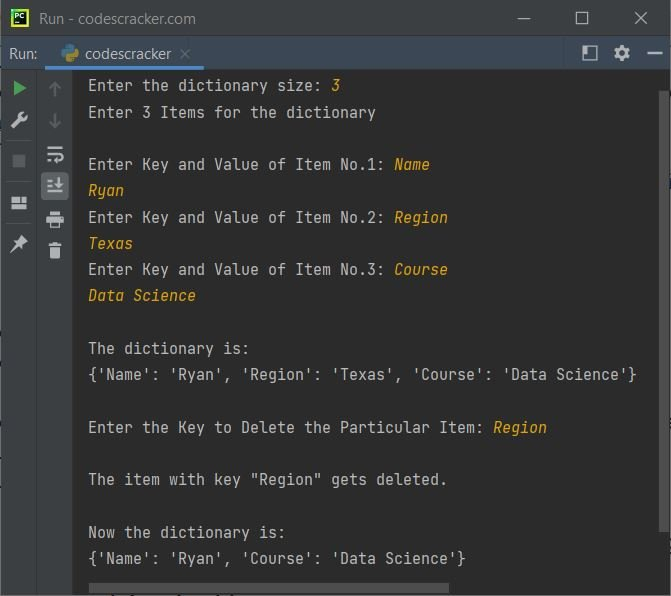Python Online Test

« Previous Function Next Function »